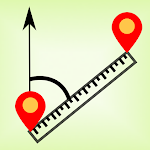5/5 - 82 votes

ID: org.project.distancecalculator

## The description of Distance & Bearing

Distance between Coordinates calculates distance between cartesien or geographic coordinates with easy-to-use interface. There are 3 methods of calculation in this app:
- Horizontal distance; calculates between two points as horizontal distance and also bearing angle as degree or grad ( between two X,Y coordinates)
- Sloped distance; calculates between two points as sloped distance, horizontal distance, height difference and bearing angle as degree or grad (between two X,Y,Z coordinates)
- Lat/Lon; calculates between two geographic locations (between two latitude and longitude coordinates). Lat/Lon calculation is based on geographiclib and the calculation result is accurate. The calculation is based on WGS84 datum.
- The app can save inputs automatically.

Distance between Coordinates has also a help page that demonstrates calculation with formulas. User can choose coordinate display as northing before easting or easting before northing. It is helpful for engineers, technicians, students and many others.